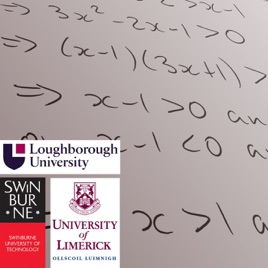300 episodes

These MathsCasts were produced by the mathematics support centres at Swinburne University, the University of Limerick and Loughborough University. They were part of a collaborative research project to develop high quality resources and investigate the effectiveness of MathsCasts to support mathematics learning. The full set of resources are available at http://commons.swin.edu.au/s/mathscasts MathsCasts are licensed under a Creative Commons Attribution-NonCommercial-NoDerivs 3.0 Unported License# MathsCasts Swinburne University of Technology

• Education

These MathsCasts were produced by the mathematics support centres at Swinburne University, the University of Limerick and Loughborough University. They were part of a collaborative research project to develop high quality resources and investigate the effectiveness of MathsCasts to support mathematics learning. The full set of resources are available at http://commons.swin.edu.au/s/mathscasts MathsCasts are licensed under a Creative Commons Attribution-NonCommercial-NoDerivs 3.0 Unported License

• video
Introduction to half range (even or odd expansion) Fourier series (MathsCasts)

## Introduction to half range (even or odd expansion) Fourier series (MathsCasts)

Introduces the idea of half-range Fourier series and addresses the question of why we use them.

• 4 min
• video
How do odd or even functions make calculation of Fourier series simpler? (MathsCasts)

## How do odd or even functions make calculation of Fourier series simpler? (MathsCasts)

Demonstrates that symmetry of functions helps us to shorten the calculation of the integrals needed for the Fourier coefficients.

• 3 min
• video
Calculating a Fourier series (MathsCasts)

## Calculating a Fourier series (MathsCasts)

Deals with the details of calculating Fourier series coefficients for a function.

• 18 min
• video
Why are circles, ellipses, parabolas and hyperbolas called 'conic sections'? Part 2 (MathsCasts)

## Why are circles, ellipses, parabolas and hyperbolas called 'conic sections'? Part 2 (MathsCasts)

We solve simultaneously the equations of a plane and a cone and show that the intersections are circles, parabolas, ellipses, hyperbolas, straight lines or just the origin.

• 11 min
• video
Sketching polynomial graphs without calculus - Part 1 (MathsCasts)

## Sketching polynomial graphs without calculus - Part 1 (MathsCasts)

Sketch of y = 2(x+1)^2-3.

• 6 min
• video
What is a Laplace transform and why do you need to study it? (MathsCasts)

## What is a Laplace transform and why do you need to study it? (MathsCasts)

Brief introduction to why we use Laplace transforms, with mention of tables.

• 4 min